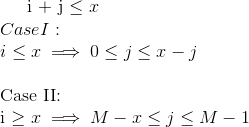# AMR14C -Editorial

#1

EASY.

Hashing

### PROBLEM:

Given an array, find out pair of numbers such their sum modulo M is less than or equal to X.

### QUICK EXPLANATION:

As the naive solution will have time complexity of O(n2) which will not pass, So we can make a count array A, where A[p] = count of numbers whose value modulo M is p and we can check if first number module M is p, then in how many ways we can select another number. The time complexity of above solution will be O(M+N).

### EXPLANATION:

Array A contains economy rates of the bowlers.

The naive solution will look like:

``````    long long int answer = 0;
for(int i =0 ; i < N ; i++)
for(int j = 0 ; i< N ; j++)
if( (A* + A[j] <= x)
answer++;
cout<<answer<<endl;
``````

But the given solution has time complexity O(n2) which will not pass in the given time limit.

As you can notice that, the value of M is not large and a solution having time complexity of O(M) will pass.

** An O(M2) approach: **

Make an array B. where

B[k] = count of numbers in array A which has value of k on taking modulo M.

Now another naive approach can be written in following manenr having time-complexity of O(M2).

``````    long long int answer = 0;
for(int i = 0 ; i< M ; i++)
for(int j = 0 ; j< M ; j++)
if( (i+j)%M <= x)
answer += B**B[j];
cout<<answer<<endl;
``````

In this approach, we are taking all such number, whole modulo M value i or j and if their sum modulo M is less than or equal to x, then they will contribute to the answer.

The above solution will also not pass, as time complexity of given solution is O(M2).

Now we will try to optimize the solution to O(M).

as we can observe that if we want ((i+j)%M) <=x, and we have fix the value of i. then j can take only few contiguous values.

There will be two cases.So, for the case I where i<=x:

``````for(int i=0 ; i<= x; i++)
answer += B**(B + B + ....+ B[x-i])
``````

As we can see that the values which we are multiplying will B*, their indices are contiguous in nature, so we can use the idea of prefix sum to get the value of the range sum in O(1) time.

Define Pre* = B + B + … + B*
then B* + … + B[x-i] = Pre[x-i] - Pre[i-1]

So modified code will look like :

``````  for(int i=0 ; i<= x; i++)
answer += B**(Pre[x-i] - Pre[i-1]);
``````

Similarly we can handle the second case when ** i>=x ** . Time complexity of the above solution will be O(M+N), which will easily pass in given time limit.

### Editorialist’s Solution:

Editorialist’s solution can be found here.

#2

I am not able to understand case 2 of I+j<=x. Please any illustration for that?

#3

Having understood the first and the second approach, I am confused and unable to understand the O(M) approach fully.

``````for (int i = 1; i<= M + X; i++)
T* = T[i-1] + T*;
long long ans = 0;
for (int i = 1; i<=n; i++)
{
if (X >= a*)
ans = ans + T[X - a*];
ans = ans + (T[M+X-a*] - T[M-1-a*]);
}
``````

Why is T being calculated up to M+X and when a*<=X why is T[M+X-a*] - T[M-1-a*] also being added to the ans?
Could you kindly explain the approach/cases in a little more detail?

Thanks.

Regards,
Ankit.

#4

This same

``````
 passes here but shows Wrong Answer at [here].
Can some help.

: http://ideone.com/FeFZ9X
: https://icpcarchive.ecs.baylor.edu/index.php?option=com_onlinejudge&Itemid=8&page=show_problem&problem=4994``````

#5

There’s bugs in both the recurrences.

1. For i<=x, You should also consider the window (M-i) <= j <= (M-1)
2. For i>x, the j is given as going till M-1. This is wrong. For example if i=x+2, and j = M-1, then (i+j)%M = (x+M+1)%M = (x+1) which is greater than x.

#6

Theres typo in editorial
In case 1: it shud be 0<=j<=x-i

#7

Bad Explanation Ever !!

#8

In the second case, i>x, So i+j can not be lesser than x. But (i+j)%M <= x which means
M<=(i+j) && (i+j)<=M+x

So M-i <=j && j <= M+x-i, as i>x
So M-i <=j && j<= M-1 [M-1 is the maximum value of M+x-i (as i>x)], Sorry for typo in latex.

#9

Thanks partner. Gotcha#10

@amitpandeykgp Please fix the solution link it’s redirecting me to the same page.

Thanks#11

Added, thanks.